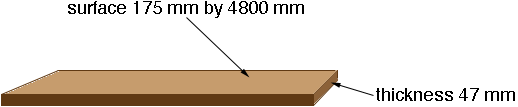SEARCH HOMEMath Central Quandaries & QueriesQuestion from remy: our timber is in square meter , our supplier is using cubic meter, how do I convert from cubic meter to square meter. Many thanks remyRemy,

There is something missing here. Square metres measures area and cubic metres measures volume and there is no conversion of one to the other.

Harley

Remy wrote back.

Hi Harley,

I have found out how my colleague "converted it". An example is: The supplier issued as this: timber 47 X175 X4800 96 pcs is 3.790 cu meters. It is converted to sq metes by : 3.790/.047/.175=460.79 sq meters. So, is this wrong then?

Remy

Hi Remy,

Now I think I understand. In this example you are ordering 96 pieces of lumber that is 4800 mm long, 175 mm wide and 47 mm thick. Maybe it is decking material. The supplier correctly calculates that this is 3.790 cu metres of lumber. You want to know how many square metres of area this will cover.

I assume you are using it flat, with the 47 mm as the thickness.If you know these dimensions you can calculate the square metres of coverage by

0.1754.8096 = 80.6 square metres

If you only know the suppliers information of 3.790 cubic metres and the thickness of 47 mm you can calculate the square metres of coverage by

3.790/0.047 = 80.6 square metres.

The calculation you supplied

3.790/.047/.175 = 460.79

is the total length of the 96 pieces in metres.

HarleyMath Central is supported by the University of Regina and The Pacific Institute for the Mathematical Sciences.